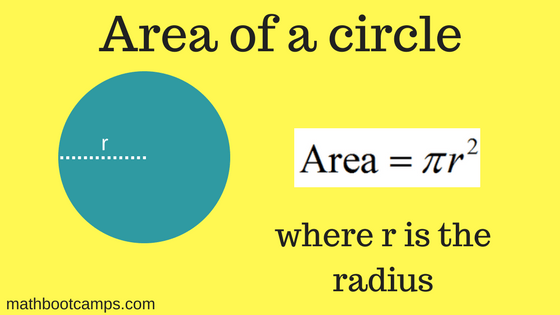# Area of a circle – formula and examples

The area of a circle can be thought of as the number of square units of space the circle occupies. This can be found using either the radius or the diameter, which we will cover in the examples below. We will also look at some examples of word problems involving area that you may come across in your studies.

## Examples of finding the area of a circle

We will use the following formula to find the area of any circle. Notice that this formula uses the radius, so we will have to convert when we are given the diameter instead. Let’s look at both cases.Find the area of a circle with a radius of 5 meters.

### Solution

Apply the formula: $$A = \pi r^2$$ with radius $$r = 5$$. Remember that $$\pi$$ is about 3.14.

\begin{align}A &= \pi (5)^2 \\ &= 25\pi \\ &\approx \bbox[border: 1px solid black; padding: 2px]{75.5 \text{ m}^2}\end{align}

Since the units of the radius were in meters, the answer is in square meters. This can be written out in words, or as $$\text{m}^2$$. Also, the final answer can be written in terms of $$\pi$$ ($$25 \pi$$ square meters) or as a decimal approximation (75.5 square meters). Which one you use depends on the application and the problem you are working on.

### Example (given diameter)

Find the area of a circle with a diameter of 6 feet.

### Solution

The radius of any circle is always half the diameter. Since the diameter of the circle is 6 feet, the radius must be 3 feet (the radius is always half of the diameter). So, we can apply the formula using $$r = 3$$.

\begin{align}A &= \pi (3)^2 \\ &= 9\pi \\ &\approx \bbox[border: 1px solid black; padding: 2px]{28.3 \text{ ft}^2}\end{align}

As you can see, it is important to pay attention to whether or not you are given the radius or the diameter of the circle. In some word problems though, this may not always be as clear.

## Word problems involving the area of a circle

Not every problem you will encounter will simply say “find the area”. In the next two examples, you will see other types of questions you might be asked.

### Example

Jason is painting a large circle on one wall of his new apartment. The largest distance across the circle will be 8 feet. Approximately how many square feet of wall will the circle cover?

### Solution

Whenever you are asked to find the number of square feet covered by something, you are finding an area. To find the area of Jason’s circle, we first need to figure out if we have been given the radius or the diameter. By definition, the diameter of a circle is the longest distance across the circle, so we know here that the diameter is 8 feet. This means that the radius is 4 feet. Therefore:

\begin{align}A &= \pi (4)^2 \\ &= 16\pi \\ &\approx \bbox[border: 1px solid black; padding: 2px]{50.2 \text{ square feet}}\end{align}

So, Jason’s circle will cover about 50.2 square feet of his wall.

### Example

The area of a circle is $$81 \pi$$ square units. What is the radius of this circle?

### Solution

To answer this question, you will have to remember a little bit of algebra. Use the formula and substitute the values you know. Then, solve for the radius, $$r$$.

$$A = \pi r^2$$

Substitute in $$A = 81 \pi$$ since you know this is the area.

$$81\pi = \pi r^2$$

Divide both sides by $$\pi$$.

$$81 = r^2$$

This can also be written as:

$$r^2 = 81$$

Take the square root to find $$r$$. Since this is a radius, the value of $$r$$ must be positive.

\begin{align}r &= \sqrt{81} \\ &= \bbox[border: 1px solid black; padding: 2px]{9}\end{align}

Therefore, the radius must be 9.